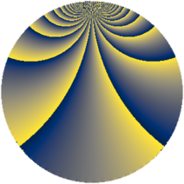# Properties

 Label 448.1.bfLevel $448$ Weight $1$ Character orbit 448.bf Rep. character $\chi_{448}(13,\cdot)$ Character field $\Q(\zeta_{16})$ Dimension $8$ Newform subspaces $1$ Sturm bound $64$ Trace bound $0$

# Related objects

## Defining parameters

 Level: $$N$$ $$=$$ $$448 = 2^{6} \cdot 7$$ Weight: $$k$$ $$=$$ $$1$$ Character orbit: $$[\chi]$$ $$=$$ 448.bf (of order $$16$$ and degree $$8$$) Character conductor: $$\operatorname{cond}(\chi)$$ $$=$$ $$448$$ Character field: $$\Q(\zeta_{16})$$ Newform subspaces: $$1$$ Sturm bound: $$64$$ Trace bound: $$0$$

## Dimensions

The following table gives the dimensions of various subspaces of $$M_{1}(448, [\chi])$$.

Total New Old
Modular forms 24 24 0
Cusp forms 8 8 0
Eisenstein series 16 16 0

The following table gives the dimensions of subspaces with specified projective image type.

$$D_n$$ $$A_4$$ $$S_4$$ $$A_5$$
Dimension 8 0 0 0

## Trace form

 $$8q + O(q^{10})$$ $$8q - 8q^{22} - 8q^{44} + 8q^{56} - 8q^{63} - 8q^{67} + 8q^{74} + O(q^{100})$$

## Decomposition of $$S_{1}^{\mathrm{new}}(448, [\chi])$$ into newform subspaces

Label Dim. $$A$$ Field Image CM RM Traces $q$-expansion
$$a_2$$ $$a_3$$ $$a_5$$ $$a_7$$
448.1.bf.a $$8$$ $$0.224$$ $$\Q(\zeta_{16})$$ $$D_{16}$$ $$\Q(\sqrt{-7})$$ None $$0$$ $$0$$ $$0$$ $$0$$ $$q-\zeta_{16}q^{2}+\zeta_{16}^{2}q^{4}+\zeta_{16}^{5}q^{7}-\zeta_{16}^{3}q^{8}+\cdots$$